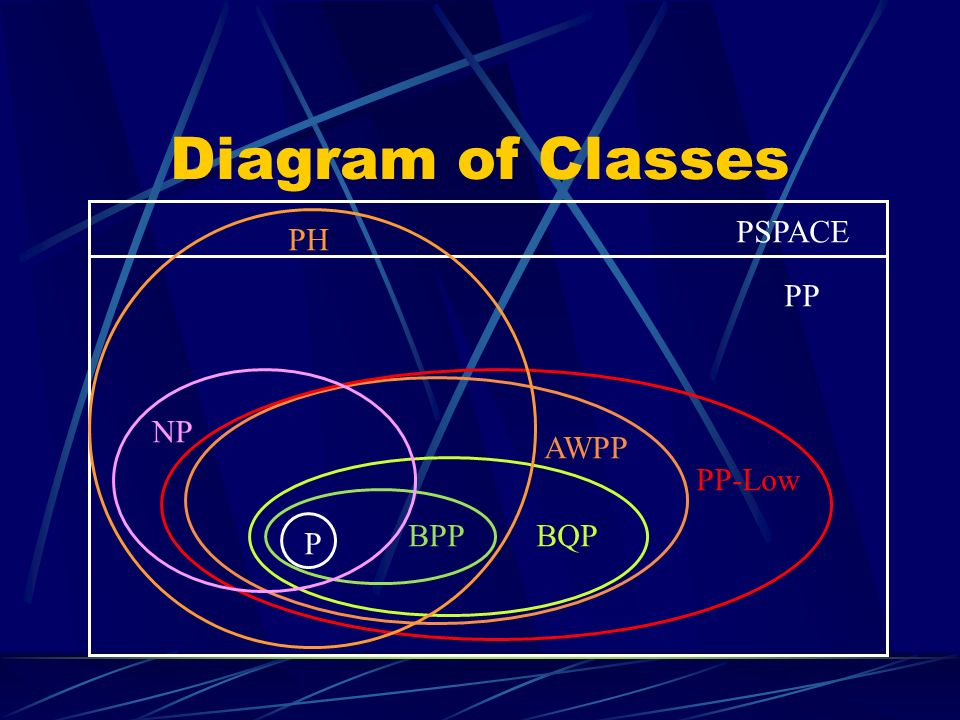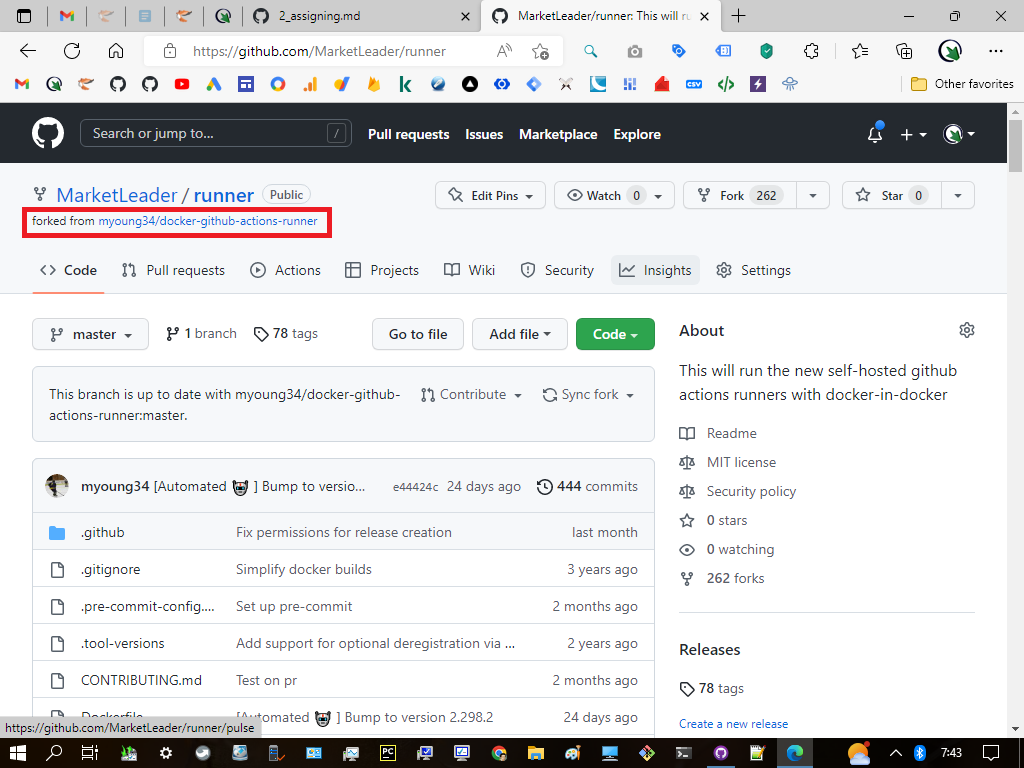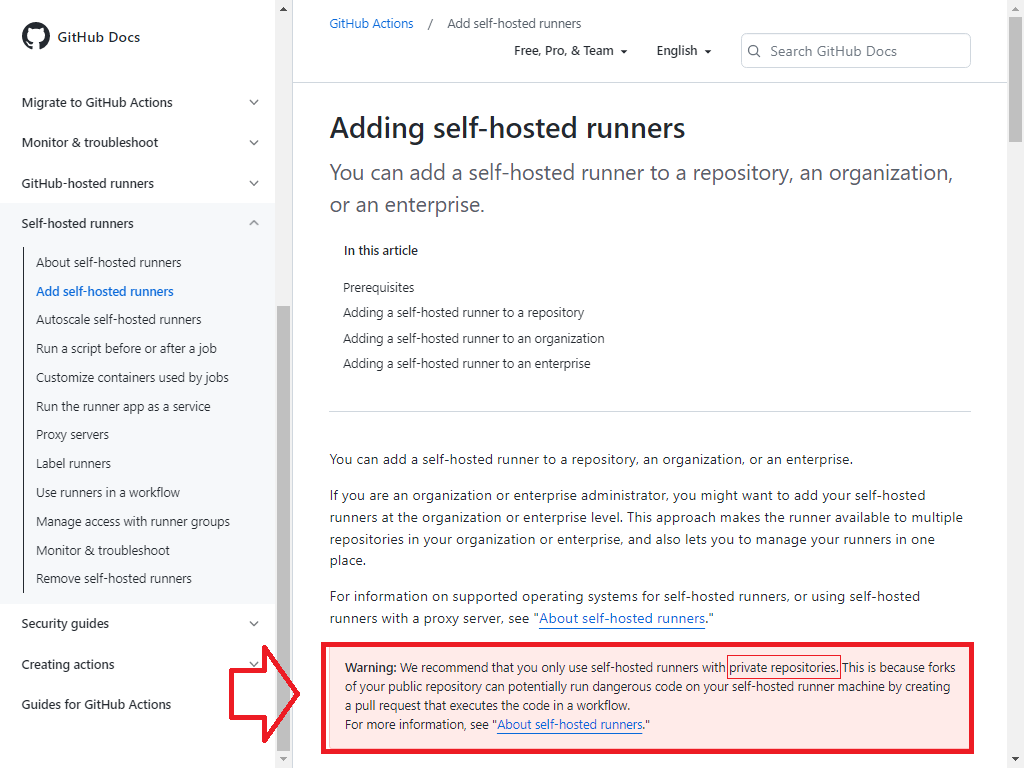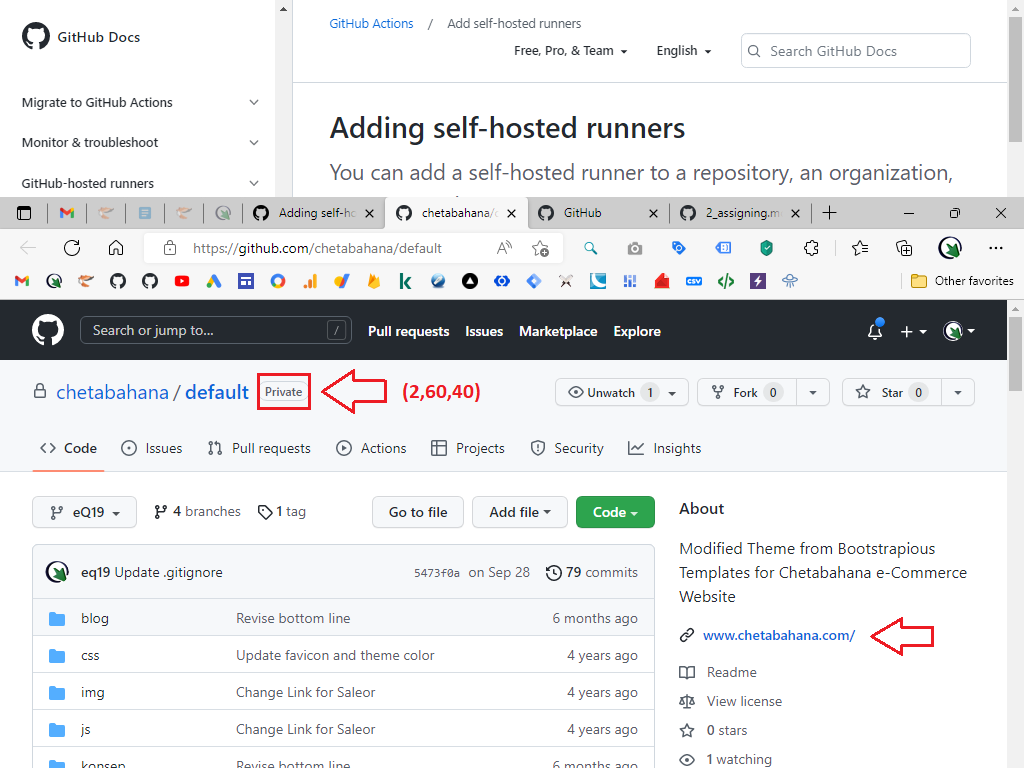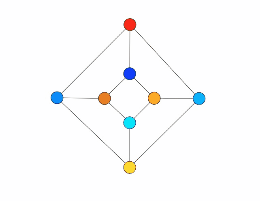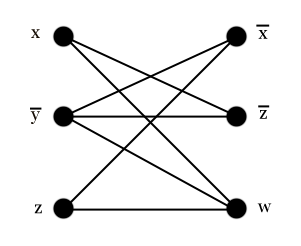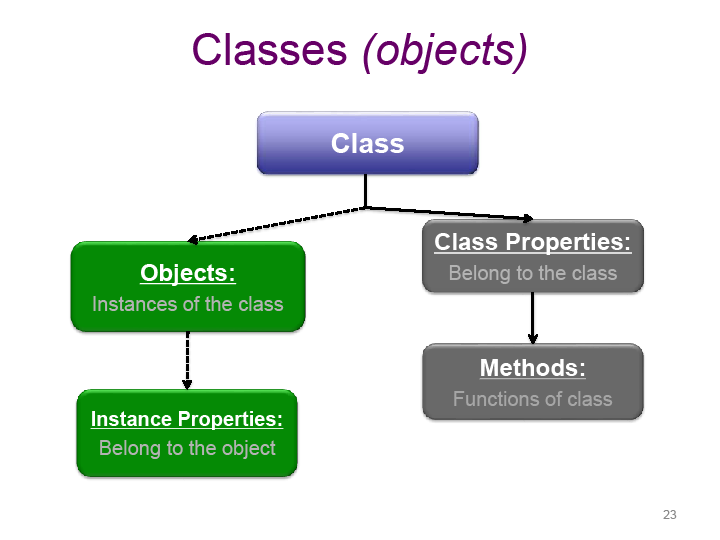This recombination scheme shall be made available on each of instance that composing 7 blocks. Since these blocks are spread in to 29 flats that bring a total of 77 rooms then the total objects per instance would become 114.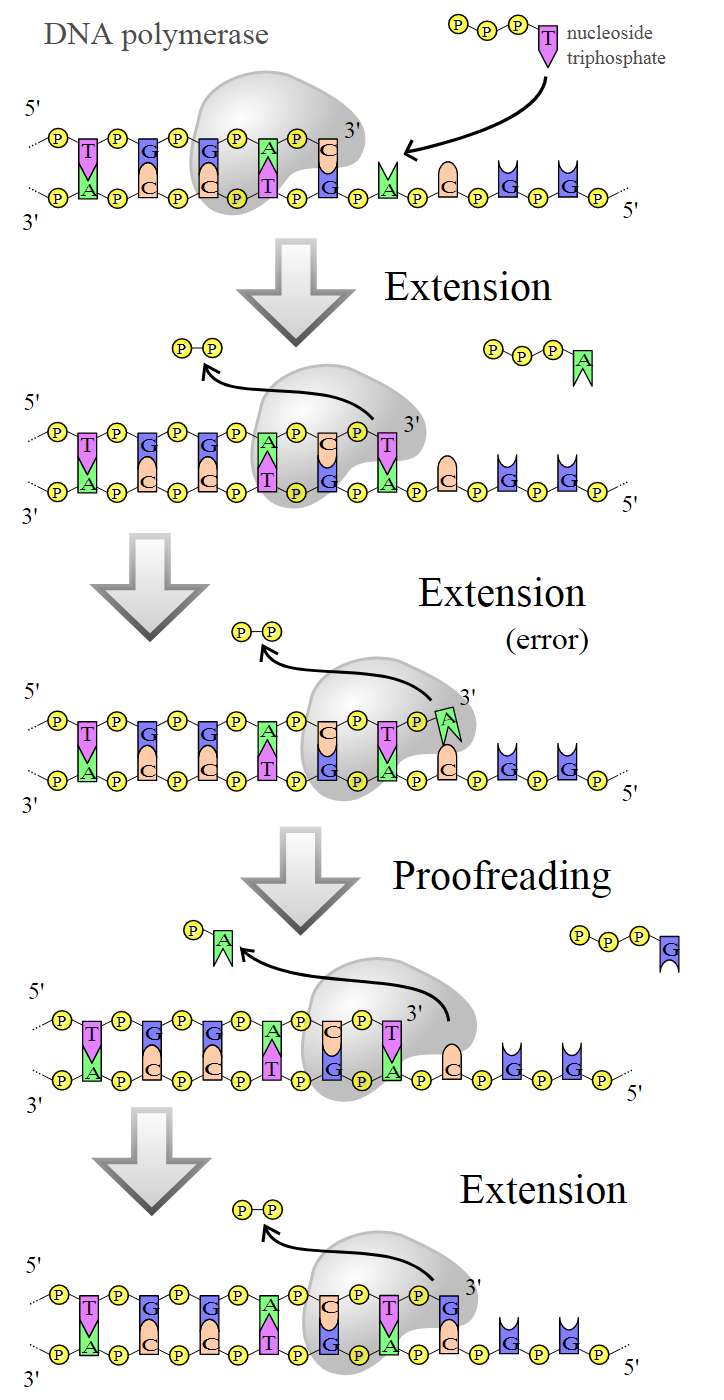Φ(13:9) = Φ(29th prime) = Φ(109) = (2+69) + 68 = 71 + 68 = 139

``````   -------------------+-----+-----+                                          ---
786 ‹------- 20:13|  90 |  90 (38) ‹-------------- ¤                      |
|                +-----+-----+                                           |
| 618 ‹- 21,22:14|   8 |  40 |  48 (40,41) ‹----------------------      17¨  class
|  |             +-----+-----+-----+-----+-----+                  |      |
|  | 594 ‹- 23:15|   8 |  40 |  70 |  60 | 100 | 278 (42) «--     |{6'®} |
|  |  |          +-----+-----+-----+-----+-----+             |    |     ---
--|--|-»24,27:16|   8 |  40 |  48 (43,44,45,46) ------------|----       |
|  |          +-----+-----+                               |           |
--|---› 28:17| 100 | {100} (50) ------------------------»           19¨  object
168        |          +-----+                                                 |
|         102 -› 29:18| 50  | 50(68) ---------> Δ18                           |
----------------------+-----+                                                ---
``````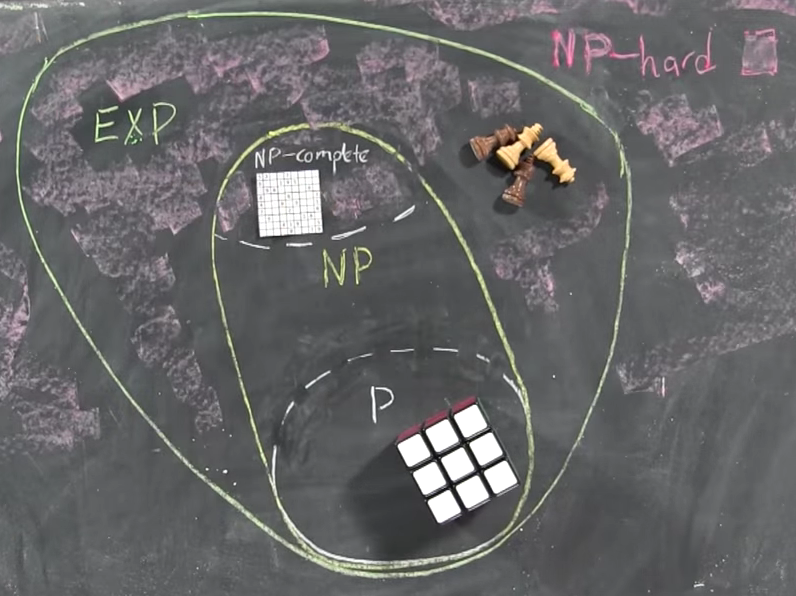In this analogy, the basic question is: is the puzzle really as hard as we think, or are we missing something? This issue was first raised by the baffling Austrian-American mathematician Kurt Gödel. It was formulated as P ≠ NP by Stephen Cook in 1971.P is a polynomial and NP is a non-deterministic polynomial. In informal terms, it asks whether every problem whose solution can be quickly verified can also be quickly solved. In simpler terms, P means a problem that is easily solved by a computer, and NP means a problem that a computer is not easy to solve but is easy to check.